# CBSE Class 10 Physics Electricity Assignment Set A

Read and download free pdf of CBSE Class 10 Physics Electricity Assignment Set A. Get printable school Assignments for Class 10 Physics. Class 10 students should practise questions and answers given here for Chapter 12 Electricity Physics in Class 10 which will help them to strengthen their understanding of all important topics. Students should also download free pdf of Printable Worksheets for Class 10 Physics prepared as per the latest books and syllabus issued by NCERT, CBSE, KVS and do problems daily to score better marks in tests and examinations

## Assignment for Class 10 Physics Chapter 12 Electricity

Class 10 Physics students should refer to the following printable assignment in Pdf for Chapter 12 Electricity in Class 10. This test paper with questions and answers for Class 10 Physics will be very useful for exams and help you to score good marks

### Chapter 12 Electricity Class 10 Physics Assignment

MULTIPLE-CHOICE QUESTIONS

Question. When electric current is passed, electrons move from:
(a) high potential to low potential.
(b) low potential to high potential.
(c) in the direction of the current.
(d) against the direction of the current.

Question. Electrical resistivity of any given metallic wire depends upon
(a) its thickness
(b) its shape
(c) nature of the material
(d) its length

Question. What is the commercial unit of electrical energy?
(a) Joules
(b) Kilojoules
(c) Kilowatt-hour
(d) Watt-hour

Question. The heating element of an electric iron is made up of:
(a) copper
(b) nichrome
(c) aluminium
(d) iron

Question. A student carries out an experiment and plots the V-I graph of three samples of nichrome wire with resistances R1 , R2 and R3 respectively. Which of the following is true?(a) R1 = R2 = R3
(b) R1 > R2 > R3
(c) R3 > R2 > R1
(d) R2 > R3 > R1

Question. In an electrical circuit two resistors of 2 Ω and 4 Ω respectively are connected in series to a 6 V battery. The heat dissipated by the 4 Ω resistor in 5 s will be
(a) 5 J
(b) 10 J
(c) 20 J
(d) 30 J

Question. Coulomb is the SI unit of:
(a) Charge
(b) current
(c) potential difference
(d) resistance

Question. Work of 14 J is done to move 2 C charge between two points on a conducting wire. What is the potential difference between the two points?
(a) 28 V
(b) 14 V
(c) 7 V
(d) 3.5 V

Question. Calculate the current flow through the 10 Ω resistor in the following circuit.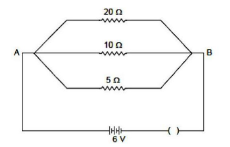(a) 1.2 A
(b) 0.6 A
(c) 0.2 A
(d) 2.0 A

Question. If R1 and R2 be the resistance of the filament of 40 W and 60 W, respectively operating 220 V, then
(a) R1 < R2
(b) R2 < R1
(c) R1 = R2
(d) R1 ≥ R2

Question. Two resistors connected in series give an equivalent resistance of 10 Ω. When connected in parallel, give 2.4 Ω. Then the individual resistance is
(a) each of 5 Ω
(b) 6 Ω and 4 Ω
(c) 7 Ω and 4 Ω
(d) 8 Ω and 2 Ω

Question. The resistance of a wire of length 300 m and cross-section area, 1.0 mm² made of material of resistivity 1.0 x 10⁻⁷ Ω is:
(a) 2 Ω
(b) 3 Ω
(c) 20 Ω
(d) 30 Ω

Question. Which of the given statements is true regarding ammeter and voltmeter?
(a) Ammeter is connected in series with the required device, Voltmeter in parallel
(b) Both ammeter and voltmeter are connected in series with required device
(c) The voltmeter is connected in series with the device, Ammeter in parallel
(d) They can be connected in any way

Question. The obstruction offered by material of conductor to the passage of electric current is known as :
(a) Resistance
(b) Conductance
(c) Inductance
(d) None of these

Question. The unit of potential difference is :
(a) Volt
(b) Ohm
(c) Ampere

Question. The instrument used for measuring electric current is :
(a) Ammeter
(b) Galvanometer
(c) Voltmeter
(d) Potentiometer

Question. While a cell is being charged, energy is converted into energy.
(a) mechanical, electrical
(b) electrical, chemical
(c) heat, electrical
(d) chemical, heat

Question. Copper is not preferred to make fuse wire because it .
(a) is a good conductor of electricity
(b) has a low melting point
(c) has a high melting point
(d) is not easily available

Question. Identify the correct circuit diagram: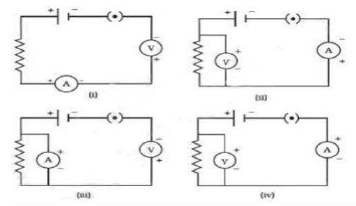(a) i
(b) ii
(c) iii
(d) iv

Question. The unit of resistivity is:
(a) V A
(b) V A
(c) V m /A
(d) VA/m

ASSERTION-REASON TYPE QUESTIONS
Following questions consist of two statements – Assertion (a) and Reason (R). Answer these questions selecting the appropriate option given below:
(a) Both A and R are true and R is the correct explanation of (a)
(b) Both A and R are true but R is not the correct explanation of (a)
(c) A is true but R is false.
(d) A is false but R is true.

Question. Assertion (a) : Longer wires have greater resistance and the smaller wires have lesser resistance.
Reason (R) : Resistance is inversely proportional to the length of the wire.-

Question. Assertion (a) : Tungsten metal is used for making filaments of incandescent lamps.
Reason (R) : The melting point of tungsten is very low.

Question. Assertion (a) : Alloys are commonly used in electrical heating devices, like electrical iron, toasters et(c)
Reason (R) : Alloys do not oxidise (burn) readily at high temperatures.

Question. Assertion (a) : Bending a wire does not affect electrical resistance.
Reason (R) : Resistance of a wire is proportional to resistivity of material.

CASE STUDY BASED QUESTIONS

1.Electrical resistivities of some substances, at 20°C are given below in the table. Study the table and answer the given questions.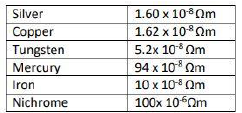Question. Which is a better conductor of electric current ?
(a) Silver
(b) Copper
(c) Tungsten
(d) Mercury

Question. Which element will be used for electrical transmission lines ?
(a) Iron
(b) Copper
(c) Tungsten
(d) mercury

Question. Nichrome is used in the heating elements of electric heating device because:
(a) It has high resistivity
(b) It does not oxidise readily at high temperature
(c) Both of the above
(d) None of the above

Question. Series arrangement is not used for domestic circuits because:
(a) Current drawn is less
(b) Current drawn is more
(c) Neither of the above
(d) Both of the above

2. In the given circuit, three identical bulbs B1, B2 and B3 are connected in parallel with a battery of 4.5 V. Study the diagram and answer the questions given below: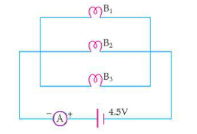Question. What will happen to the other two bulbs if the bulb B3 gets fused ?
(a) They will also stop glowing.
(b) Other bulbs will glow with same brightness.
(c) They will glow with low brightness.
(d) They glow with more brightness.

Question. If the wattage of each bulb is 1.5 W, how much readings will the ammeter A show when all the three bulbs glow simultaneously?
(a) 1.1 A
(b) 2.1 A
(c) 1.5 A
(d) None of the above

Question. Find the total resistance of the circuit.
(a) 1.0 Ω
(b) 4.1 Ω
(c) 1.5 Ω
(d) 2.0 Ω

Question. How many resistors of 88 Ω are connected in parallel to carry 10 A current on a 220 V line ?
(a) 2 resistors
(b) 1 resistors
(c) 3 resistors
(d) 4 resistors

Question : What does an electric circuit mean?
Answer- A continuous closed path made of electric components through which an electric current flows is known as an electric circuit.

Question : Define the unit of current.
Answer- The unit of current is ampere. Ampere is defined by the flow of one coulomb of charge per second.

Question : Calculate the number of electrons constituting one coulomb of charge.
Answer- Charge = q = 1 C
Number of electrons = n = ?
Charge on an electron = e = 1.6 x 10-19 C
We know that, q = ne
Hence, n = q/e = 1 / 1.6 x 10-19 = 6.25 x 1018 electrons.

Question : Name a device that helps to maintain a potential difference across a conductor.

Question : What is meant by saying that the potential difference between two points is 1 V?
Answer- When 1 J of work is done to move a charge of 1 C from one point to another, it is said that the potential difference between two points is 1 V.

Question : How much energy is given to each coulomb of charge passing through a 6 V battery?
Answer- Charge = q = 1 C
Potential difference = V = 6 V
Energy = W = ?
We know that, v =w/q
, Hence, W = Vq = 6 x 1 = 6 J (joule).

Question : On what factors does the resistance of a conductor depend?
Answer- The resistance of the conductor depends on: Length, Cross-sectional area, Temperature and Nature of the material of the conductor.
(1) Resistance is directly proportional to the length of conductor.
(2) Resistance is inversely proportional to the area of cross section of the conductor.

Question : Will current flow more easily through a thick wire or a thin wire of the same material, when connected to the same source? Why?
Answer- The current will flow more easily through thick wire. It is because the resistance of a conductor is inversely proportional to its area of cross - section. So thicker wire has less resistance and hence more easily the current flows.

Question : Let the resistance of an electrical component remain constant while the potential difference across the two ends of the component decreases to half of its former value. What change will occur in the current through it?
Answer- According to Ohm’s law (V=IR) current is directly proportional to the potential difference across the ends of the conductor if resistance remains constant. Hence current will also decrease to half of its former value
i.e.1/2.

Question : Why are coils of electric toasters and electric irons made of an alloy rather than a pure metal?
Answer- Because the melting point and resistivity of an alloy are much higher than a pure metal.

Question : Use the data in the table 12.2 and answer the following questions: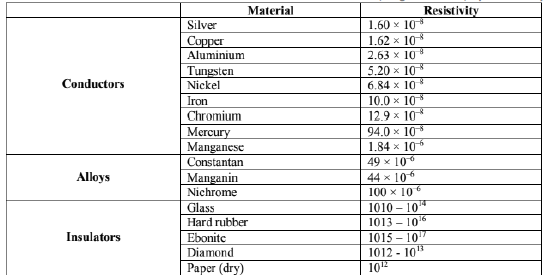(a): Which among iron and mercury is a better conductor?

(b): Which material is the best conductor?

Question : Draw a schematic diagram of a circuit consisting of a battery of three cells of 2 V each, a 5 Ω resistor, an 8 Ω resistor, and a 12 Ω resistor, and a plug key, all connected in series.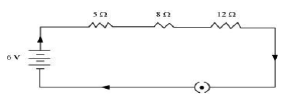Question : Redraw the circuit of Question 12, putting in an ammeter to measure the current through the resistors and a voltmeter to measure the potential difference across the 12 Ω resistor. What would be the readings in the ammeter and the voltmeter?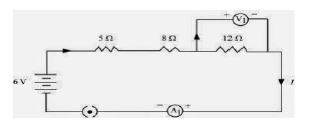R1 = 5 Ω, R2 = 8 Ω, R3 = 12 Ω
As resistors are combines in series, So total resultant resistance is:
R = R1 + R2 + R3 = 5 + 8 + 12 = 25 Ω
Now total current or reading of ammeter = I =  V/R =6/25 = 0.24 A
Now for 12 Ω Resistor, Reading of voltmeter = V = IR = 0.24 x 12 = 2.88 V.

Question : Judge the equivalent resistance when the following are connected in parallel – (a) 1 Ω and 106 Ω, (b) 1 Ω, 103 Ω, and 106 Ω.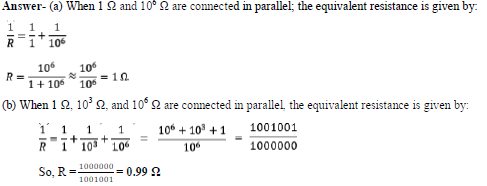Question : An electric lamp of 100 Ω, a toaster of resistance 50 Ω, and a water filter of resistance 500 Ω are connected in parallel to a 220 V source. What is the resistance of an electric iron connected to the same source that takes as much current as all three appliances, and what is the current through it?
Answer- Circuit diagram for the given appliances will be as:Now equivalent resistance (R) for the circuit is: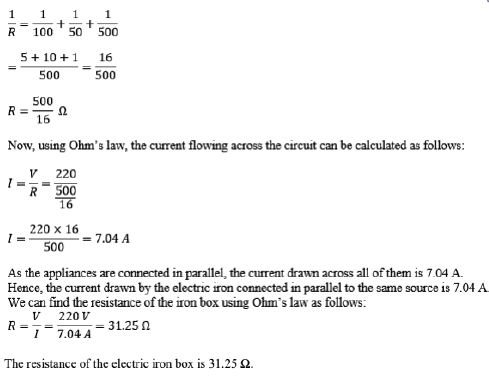Question : What are the advantages of connecting electrical devices in parallel with the battery instead of connecting them in series?
Answer- (1) Parallel connection of devices reduces the effective resistance of the circuit.
(2) In parallel connection each device needs same voltage.
(3) Also in parallel connection if one device stops working it will not affect others.

Question : How can three resistors of resistances 2 Ω, 3 Ω, and 6 Ω be connected to give a total resistance of (a) 4 Ω, (b) 1 Ω?
Answer- (a) The circuit diagram below shows the connection of three resistors to get a total resistance of 4Ω: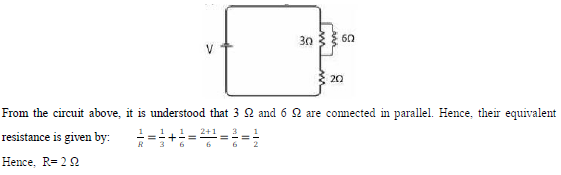The equivalent resistor 2 Ω is in series with the 2 Ω resistor. Now the equivalent resistance can be calculated as follows: Req= 2 Ω +2 Ω = 4 Ω
(b) The circuit diagram below shows the connection of three resistors to get a total resistance of 1 Ω: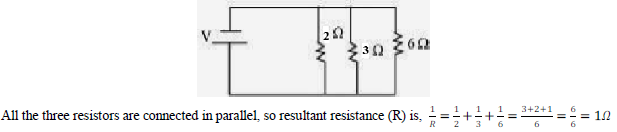Question 18: What is (a) the highest, (b) the lowest total resistance that can be secured by combinations of four coils of resistance 4 Ω, 8 Ω, 12 Ω, 24 Ω?
Solution: (a) In series combination, the highest resistance will be obtained,
R = 4 Ω + 8 Ω + 12 Ω + 24 Ω = 48 Ω.
(b) In parallel combination, the lowest resistance will be obtained,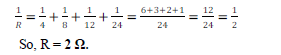Question : Why does the cord of an electric heater not glow while the heating element does?
Answer- The heating element of an electric heater is made of an alloy which has a high resistance. When the current flows through the heating element, the heating element becomes too hot and glows red. The cord is usually made of copper or aluminum which has low resistance. Hence the cord doesn’t glow.

Question : Compute the heat generated while transferring 96000 coulomb of charge in one hour through a potential difference of 50 V.
Answer- Charge = q = 96000 C
Time = t = 1 hour = 3600 sec
Potential difference = V = 50 V
Heat generated = W = q x V = 96000 x 50 = 4800000 = 4.8 x 106 Joules.

Question : An electric iron of resistance 20 Ω takes a current of 5A.Calculate the heat developed in 30 s.
Answer- Resistance = R = 20 Ω,
Current = I = 5 A,
Time = t = 30 sec
Heat = W = I2Rt = (5)2 x 20 x 30 = 25 x 600 = 15000 joules.

Question : What determines the rate at which energy is delivered by a current?

Question : An electric motor takes 5 A from a 220 V line. Determine the power of the motor and the energy consumed in 2 h.
Answer- Current = I = 5 A,
Pot. Diff. = V = 220 V,
Time = t = 2 h
Power = P = VI = 5 x 220 = 1100 W (watt) = 1.1kW
Energy = W = P x t = 1.1 x 2 kWh = 2.2 kWh

More Question-

1. When does the current flow in an electric circuit?
2. What is the difference between resistance and resistor?
3. What are the factors on which the resistance of conductor depends? Give the corresponding relation.
4. Calculate the resistance of a 2m long nichrome wire of radius 0.321mm. Resistivity of nichrome is 15 x 10-6 Ω m. If the potential difference of 10v is applied across this wire, what will be the current in the wire?
5. Derive an expression for the equivalent resistance of three resistances connected in series?
6. Derive an expression for the combination of three resistances connected in parallel.
7. Express Ohm’s law both by a mathematical formula and by a graph.
8. Derive an expression for the heat produced in a resistor R when a voltage drop across it is V. Hence state Joule’s law of heating.
9. Describe some practical applications of heating effect of the electric current.
10. An electrical heater is used on a 220v supply and takes a current of 5A
1. What is its power
2. What is the cost of using the heater for 50 hours if 1 KWh costs Rs. 1.50?
11. A house hold uses the following electric appliances :
i) Refrigerator of rating 400 W for 10 hours each day.
ii) Two electric fans of rating 80 W each for 12 hours each day.
iii) Six electric tubes of rating 18 W each for 6 hours each day.
Calculate the electricity bill of the house hold for the month of June if the cost per unit of electric energy is Rs. 3
12. Distinguish between kilowatt and kilowatt hour.
13. Define the term electric energy. Write an expression of the electric energy consumed in an electric circuit.
14. Define the term electric power. Write an expression for it.
15. Define kilowatt hour. How many joules are equal to 1 KWh.
16. Two identical resistors, each of resistance 10 Ohms are connected
i) in series ii) in parallel, in turns to a battery of 6 volts. Calculate the ratio of power consumed in the combination of resistors in two cases.

Please click the below link to access CBSE Class 10 Physics Electricity Assignment Set A

 CBSE Class 10 Science Chemical Reactions and Equations Assignment
 CBSE Class 10 Science Acids Bases and Salts Assignment Set A CBSE Class 10 Science Acids Bases and Salts Assignment Set B CBSE Class 10 Science Acids Bases and Salts Assignment Set C
 CBSE Class 10 Science Metals and Non Metals Assignment Set A CBSE Class 10 Science Metals and Non Metals Assignment Set B
 CBSE Class 10 Science Carbon and its compounds Assignment Set A CBSE Class 10 Science Carbon and its compounds Assignment Set B CBSE Class 10 Science Carbon and its compounds Assignment Set C CBSE Class 10 Science Carbon and its compounds Assignment Set D CBSE Class 10 Science Carbon and its compounds Assignment Set E
 CBSE Class 10 Science Periodic Classification Of Elements Assignment Set A CBSE Class 10 Science Periodic Classification Of Elements Assignment Set B CBSE Class 10 Science Periodic Classification Of Elements Assignment Set C
 CBSE Class 10 Science Biology Life Process Respiration Assignment CBSE Class 10 Science Biology Nutrition Assignment CBSE Class 10 Science Biology Respiration Assignment CBSE Class 10 Science Biology Transport Enrichment Assignment CBSE Class 10 Science Biology Transportation Assignment CBSE Class 10 Science Life Processes Assignment Set A CBSE Class 10 Science Life Processes Assignment Set B CBSE Class 10 Science Life Processes Assignment Set C
 CBSE Class 10 Science Control and Coordination Assignment Set A CBSE Class 10 Science Control and Coordination Assignment Set B CBSE Class 10 Science Control and Coordination Assignment Set C CBSE Class 10 Science Control and Coordination Assignment Set D
 CBSE Class 10 Science How do Organisms Reproduce Assignment Set A CBSE Class 10 Science How do Organisms Reproduce Assignment Set B
 CBSE Class 10 Science Heredity and Evolution Assignment Set A CBSE Class 10 Science Heredity and Evolution Assignment Set B CBSE Class 10 Science Heredity and Evolution Assignment Set C
 CBSE Class 10 Science Light Reflection and Refraction Assignment Set A CBSE Class 10 Science Light Reflection and Refraction Assignment Set B CBSE Class 10 Science Light Reflection and Refraction Assignment Set C CBSE Class 10 Science Light Reflection and Refraction Assignment Set D CBSE Class 10 Science Light Reflection and Refraction Assignment Set E CBSE Class 10 Science Light Reflection and Refraction Assignment Set F
 CBSE Class 10 Science Human Eye and Colorful World Assignment Set A CBSE Class 10 Science Human Eye and Colorful World Assignment Set B CBSE Class 10 Science Human Eye and Colorful World Assignment Set C
 CBSE Class 10 Physics Electricity Assignment Set A CBSE Class 10 Physics Electricity Assignment Set B CBSE Class 10 Physics Electricity Assignment Set C CBSE Class 10 Science Electricity Assignment Set A CBSE Class 10 Science Electricity Assignment Set B CBSE Class 10 Science Electricity Assignment Set C
 CBSE Class 10 Science Magnetic Effects of Electric Current Assignment Set A CBSE Class 10 Science Magnetic Effects of Electric Current Assignment Set B CBSE Class 10 Science Magnetic Effects of Electric Current Assignment Set C CBSE Class 10 Science Magnetic Effects of Electric Current Assignment Set D
 CBSE Class 10 Science Sources of Energy Assignment Set A CBSE Class 10 Science Sources of Energy Assignment Set B CBSE Class 10 Science Sources of Energy Assignment Set C CBSE Class 10 Science Sources of Energy Assignment Set D
 CBSE Class 10 Science Our Environment Assignment Set A CBSE Class 10 Science Our Environment Assignment Set B CBSE Class 10 Science Our Environment Assignment Set C
 CBSE Class 10 Science Sustainable Management of Natural Resources Assignment Set A CBSE Class 10 Science Sustainable Management of Natural Resources Assignment Set B CBSE Class 10 Science Sustainable Management of Natural Resources Assignment Set C
 CBSE Class 10 Physics Revision Assignment Set A CBSE Class 10 Physics Revision Assignment Set B CBSE Class 10 Physics Revision Assignment Set C CBSE Class 10 Physics Revision Assignment Set D CBSE Class 10 Physics Revision Assignment Set E CBSE Class 10 Physics Revision Assignment Set F CBSE Class 10 Physics Revision Assignment Set G CBSE Class 10 Physics Revision Assignment Set H
 CBSE Class 10 Science Assignment CBSE Class 10 Science Assignments Collection CBSE Class 10 Science Energy Crossword Puzzle Assignment
 CBSE Class 10 Physics Question Bank CBSE Class 10 Physics Tungsten Assignment
 CBSE Class 10 Science Chemistry Cleaning Capacity of Soap Assignment CBSE Class 10 Science Chemistry Determination of pH Assignment CBSE Class 10 Science Chemistry Different Types of Chemical Reactions Assignment CBSE Class 10 Science Chemistry Displacement Reaction and Reactivity Series Assignment CBSE Class 10 Science Chemistry Properties of Acetic Acid Assignment CBSE Class 10 Science Chemistry Saponification Preparation of Soap Assignment CBSE Class 10 Science Chemistry Study of properties of HCI Acid and NaOH Assignment

## More Study Material

Tags

### CBSE Class 10 Physics Chapter 12 Electricity Assignment

We hope you liked the above assignment for Chapter 12 Electricity which has been designed as per the latest syllabus for Class 10 Physics released by CBSE. Students of Class 10 should download and practice the above Assignments for Class 10 Physics regularly. We have provided all types of questions like MCQs, short answer questions, objective questions and long answer questions in the Class 10 Physics practice sheet in Pdf. All questions have been designed for Physics by looking into the pattern of problems asked in previous year examinations.

#### Assignment for Physics CBSE Class 10 Chapter 12 Electricity

Our team of expert teachers have referred to NCERT book for Class 10 Physics to design the Physics Class 10 Assignments. If you practice at least one test paper daily, you will get higher marks in Class 10 exams this year. Daily practice of Physics course notes and related study material will help you to clear all your doubts and have stronger understanding of all concepts. You can download all Revision notes for Class 10 Physics also from www.studiestoday.com absolutely free of cost.

#### Chapter 12 Electricity Assignment Physics CBSE Class 10

All questions and their answers for the assignment given above for Class 10 Physics have been developed as per the latest curriculum and books issued for the current academic year. The students of Class 10 can rest assured that the best teachers have designed the questions of Physics so that you are able to revise the entire syllabus if you do the assignments. Lot of MCQ questions for Class 10 Physics have also been given in the worksheets and assignments for regular use. All study material for Class 10 Physics students have been given on studiestoday.

#### Chapter 12 Electricity Assignment CBSE Class 10 Physics

Regular assignment practice helps to get a more comprehensive understanding of Chapter 12 Electricity concepts. Assignments play a crucial role in understanding Chapter 12 Electricity in CBSE Class 10. Students can download all the assignments of the same chapter in Class 10 Physics in Pdf format. You can print them or read them online on your computer or mobile.

#### CBSE Physics Class 10 Chapter 12 Electricity Assignment

CBSE Class 10 Physics latest books have been used for coming up with the latest questions and solutions for the above assignment. If you have revised all concepts relating to Chapter 12 Electricity then you should attempt all questions given in the test sheets above. We have also provided lot of Worksheets for Class 10 Physics which you can use to further make your self stronger in Physics

Where can I download in PDF assignments for CBSE Class 10 Physics Chapter 12 Electricity

You can download free Pdf assignments for CBSE Class 10 Physics Chapter 12 Electricity from StudiesToday.com

The assignments for Chapter 12 Electricity Class 10 Physics for have been made based on which syllabus

The Chapter 12 Electricity Class 10 Physics Assignments have been designed based on latest CBSE syllabus for Class 10 Physics issued for the current academic year

Can I download and print these printable assignments for Physics Chapter 12 Electricity Class 10

Yes, These printable assignments for Chapter 12 Electricity Class 10 Physics are free to download and print

How many topics are covered in Chapter 12 Electricity Physics assignments for Class 10

All topics given in Chapter 12 Electricity Physics Class 10 Book for the current academic year have been covered in the given assignment

Is there any charge for this assignment for Chapter 12 Electricity Physics Class 10

No, all Printable Assignments for Chapter 12 Electricity Class 10 Physics have been given for free and can be downloaded in Pdf format

How can I download the printable test assignments for Chapter 12 Electricity Physics Class 10

Just click on the View or Download button below, then another window with the Pdf will be visible, just click on the Pdf icon to download the free assignments for Chapter 12 Electricity Class 10 Physics

Are these assignments available for all chapters in Class 10 Physics

Yes, apart from Physics you can download free assignments for all subjects in Class 10

Can I download solved assignments for Chapter 12 Electricity CBSE Class 10 Physics

Our team of expert teachers at studiestoday.com have provided all answers for the practice questions which have been given in Class 10 Physics Chapter 12 Electricity assignments